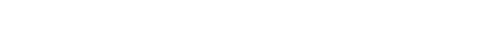glGetClipPlane.3glOpenGL man pages

```

```

## Name

```  glGetClipPlane - return the coefficients of the specified clipping plane

```

## C Specification

```  void glGetClipPlane( GLenum plane,
GLdouble	*equation )

```

## Parameters

```
plane	    Specifies a	clipping plane.	 The number of clipping	planes
depends on the implementation, but at least	six clipping planes
are	supported.  They are identified	by symbolic names of the form
GL_CLIP_PLANEi where 0 <= i < GL_MAX_CLIP_PLANES.

equation  Returns four double-precision values that are the coefficients of
the	plane equation of plane	in eye coordinates.

```

## Description

```  glGetClipPlane returns in equation the four coefficients of the plane
equation for plane.

```

## Notes

```  It is	always the case	that GL_CLIP_PLANEi = GL_CLIP_PLANE0 + i.

If an	error is generated, no change is made to the contents of equation.

```

## Errors

```  GL_INVALID_ENUM is generated if plane	is not an accepted value.

GL_INVALID_OPERATION is generated if glGetClipPlane is executed between the
execution of glBegin and the corresponding execution of glEnd.

```

```  glClipPlane